# Identify the Missing Symbol to Complete the 4 Box Grid

In this worksheet, students will find the missing symbol to complete a grid of four boxes. It will develop their ability to find and follow patterns in shapes and symbols.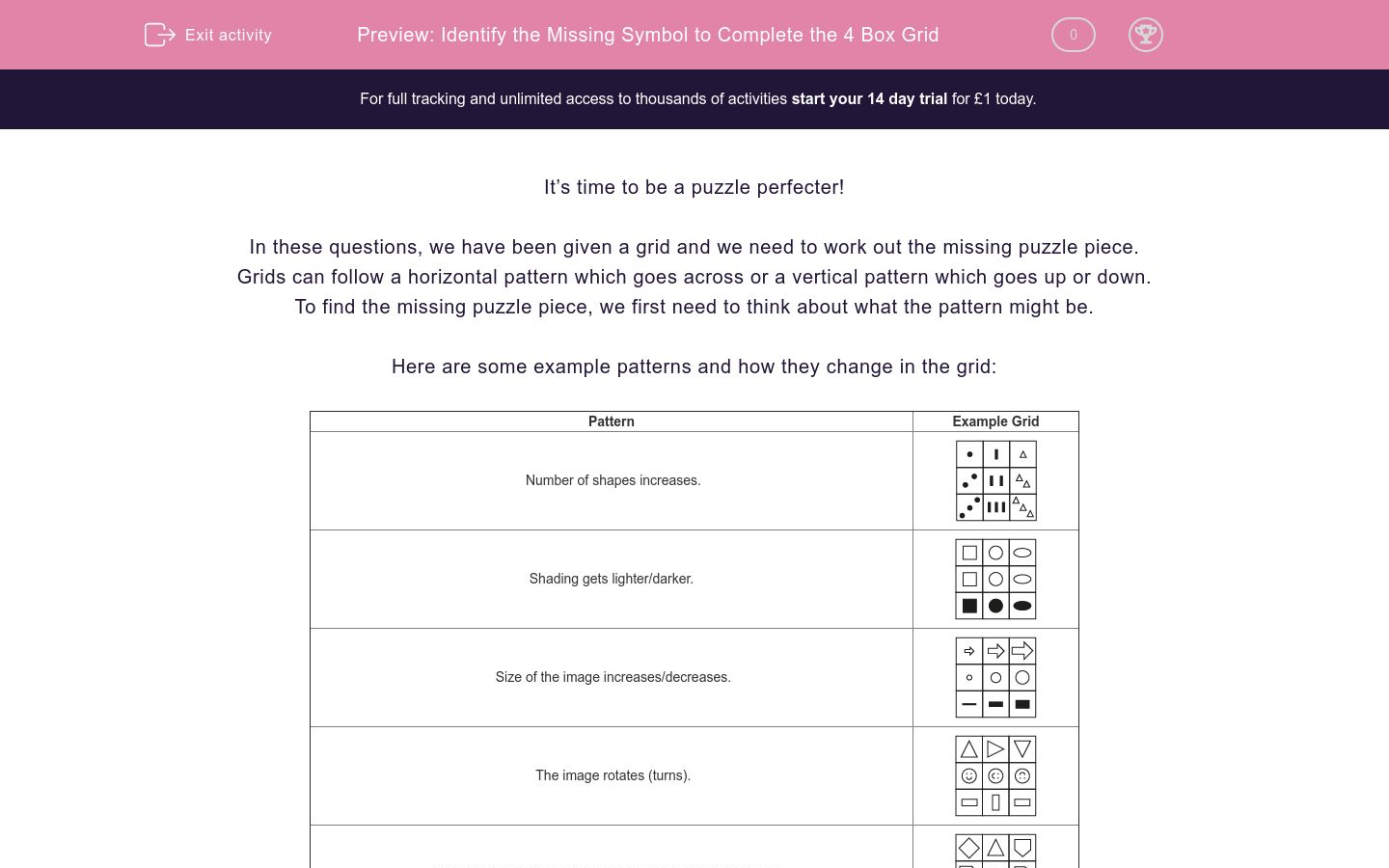Key stage:  KS 2

Curriculum topic:   Spatial and Non-Verbal Reasoning

Curriculum subtopic:   Complete the Grid

Difficulty level:### QUESTION 1 of 10

It’s time to be a puzzle perfecter!

In these questions, we have been given a grid and we need to work out the missing puzzle piece.

Grids can follow a horizontal pattern which goes across or a vertical pattern which goes up or down.

To find the missing puzzle piece, we first need to think about what the pattern might be.

Here are some example patterns and how they change in the grid:

 Pattern Example Grid Number of shapes increases.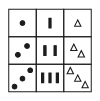Shading gets lighter/darker.Size of the image increases/decreases.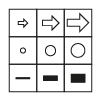The image rotates (turns).The number of sides of the shapes in the image increases.Take a look at this example:Which of the following images would complete this grid?
a)b)c)d)The pattern in this case is horizontal.

In each square of the grid, the number of sides of each shape increases by one each time. So the answer is c!

It’s now your turn to find the missing puzzle piece in grids.

Remember to decide if it is horizontal or vertical when working out what the pattern is. Good luck puzzle champ!

Always rule out any obviously incorrect answers.

Identify ALL the statements that describe why option b is the INCORRECT answer.b)The triangles are black.

They are the the wrong type of triangles.

The triangles are not an upside down image of the box above.

The triangles are pointing upwards instead of downwards.

Complete the grid below with the image that best fits in the space.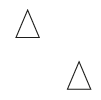Complete the grid below with the image that best fits in the blank space.Identify ALL the statements that describe why option c is the INCORRECT answer.c)The image is white.

It should be a circle not a triangle.

This is a translation of the image on the bottom right rather than a reflection.

It is the wrong type of triangle.

Complete the grid below with the image that best fits in the blank space.Identify ALL the statements that describe why option b is the INCORRECT answer.b)The pentagon is white.

The star is black.

This is a translation of the image in the bottom right rather than a reflection.

The shape has the wrong orientation.

Complete the grid below with the image that best fits in the blank space.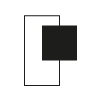Identify ALL the statements that describe why option a is the INCORRECT answer.a)The rectangle is white.

The square is black.

This is not a mirror reflection.

The shape has the wrong orientation.

Complete the grid below with the image that best fits in the blank space.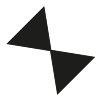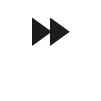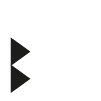Identify ALL the statements that describe why option b is the INCORRECT answer.b)The triangles are pointing downwards.

The traingles are the wrong size.

The triangles are the wrong colour.

The triangles are not aligned centrally.

• Question 1

Identify ALL the statements that describe why option b is the INCORRECT answer.b)The triangles are black.
They are the the wrong type of triangles.
The triangles are not an upside down image of the box above.
The triangles are pointing upwards instead of downwards.
EDDIE SAYS
The triangles should be white so option a is incorrect. The triangles should not be right-angled triangles so option b is incorrect. The triangles are not pointing downwards like they are in the left-hand column so option c is not correct
• Question 2

Complete the grid below with the image that best fits in the space.EDDIE SAYS
Let's approach this one step by step like an expert detective. In the missing square of the grid, we would expect to see two triangles and we would expect them to be white (to follow the pattern from the square above). This means we can rule out option b straight away. We would also expect the two triangles to be facing downwards as this is how the pattern is in the first column. This means the only possible correct option is c). Did your detective skills spot the clues to help you find the right answer?
• Question 3

Complete the grid below with the image that best fits in the blank space.EDDIE SAYS
Let's make some initial observations here. In the top row, we have two images that look exactly alike but they are actually a mirror image of each other, so we would expect the bottom row to follow a similar pattern. We are looking for a mirror image of the bottom right corner. This makes option d the best fit here.
• Question 4

Identify ALL the statements that describe why option c is the INCORRECT answer.c)This is a translation of the image on the bottom right rather than a reflection.
EDDIE SAYS
There is only one thing wrong here and that is that this image is a translation rather than a reflection. Have you heard of the term translation before? It means that the shape is exactly the same, but it has been moved along rather than flipped or rotated, which is what we were looking for in this question.
• Question 5

Complete the grid below with the image that best fits in the blank space.EDDIE SAYS
This is another example where reflection has been used. We can see that the two triangles are mirror images of each other. What does the black shape look like when it is reflected? It would be pointing towards the top right-hand corner. So options b and c can be ruled out on this information alone. Options a and d match this description and look very similar. Did you spot the different positions of the star? In option a the top point of the star is facing upwards and to the right in the same way it is in the first column. This makes option a the correct answer.. Very well done indeed if your detective skills picked up on that small difference!
• Question 6

Identify ALL the statements that describe why option b is the INCORRECT answer.b)The pentagon is white.
The star is black.
The shape has the wrong orientation.
EDDIE SAYS
Option b doesn't fit because the colours match the left-hand side of the grid rather than fitting with the image in the bottom right. It isn't a translation - it is a rotation and, in this case, it also has the wrong orientation. Great justification work grid detective!
• Question 7

Complete the grid below with the image that best fits in the blank space.EDDIE SAYS
Did you spot that option b was the best fit here grid detective? First, let's look at the left side of the grid. The shapes are the same but the image has been reflected. Imagine that the central horizontal line of the grid is a mirror - can you see that the top has been reflected into the bottom and vice versa? So on the right, we are looking for an image that is the same as the bottom image but has also been reflected in the same way. The key thing to spot here is that the black square is positioned lower down on the right, so the missing image will inverse this and have the black square positioned higher up. This makes option b the right answer.
• Question 8

Identify ALL the statements that describe why option a is the INCORRECT answer.a)This is not a mirror reflection.
The shape has the wrong orientation.
EDDIE SAYS
Option a is incorrect because it has the wrong orientation. It is also not a mirrored reflection of the grid. You'll see this if we placed a mirror on the middle horizontal line. Let's be more technical while we're on the topic. Have you ever heard about the x and y axis? The x axis is the horizontal line which goes from left to right in this grid. The y axis is the vertical line which goes from north to south in this grid. In this grid, we needed to reflect the shape in the bottom, right-hand corner in the x axis, which is why option a is incorrect too. If we were reflecting it in the y axis (vertical), then this would be a correct mirror reflection so this is a true statement.
• Question 9

Complete the grid below with the image that best fits in the blank space.EDDIE SAYS
Does this feel like it's getting a bit trickier? Don't worry detective, it is! Let's describe what we have here. On the top left, we have two large triangles facing each other and meeting at their tips, one pointing upwards and one pointing downwards. In the top right, we have two smaller triangles both facing upwards. In the bottom right, we have two large triangles meeting at the tip again but pointing left and right. So our first thought is that we need an option which has two smaller triangles, which excludes option a. Now let's think about the direction of the arrows. We need the two small triangles to be pointing sideways to link with the bottom right corner. This leaves a choice of c and d. Option d is not the same shape as the two small triangles at the top so the option that matches this pattern is option c.
• Question 10

Identify ALL the statements that describe why option b is the INCORRECT answer.b)The triangles are pointing downwards.
EDDIE SAYS
Did you spot that there is just one reason why option b doesn't fit well in the grid? The arrows are pointing downwards and we were looking for arrows that are facing sideways to fit with the rest of the pattern. Everything else about option b matches with the correct answer, which shows us why it is SO important to work through the options and rule them out as you spot a feature that doesn't fit with the pattern you have discovered.
---- OR ----

Sign up for a £1 trial so you can track and measure your child's progress on this activity.

### What is EdPlace?

We're your National Curriculum aligned online education content provider helping each child succeed in English, maths and science from year 1 to GCSE. With an EdPlace account you’ll be able to track and measure progress, helping each child achieve their best. We build confidence and attainment by personalising each child’s learning at a level that suits them.

Get started